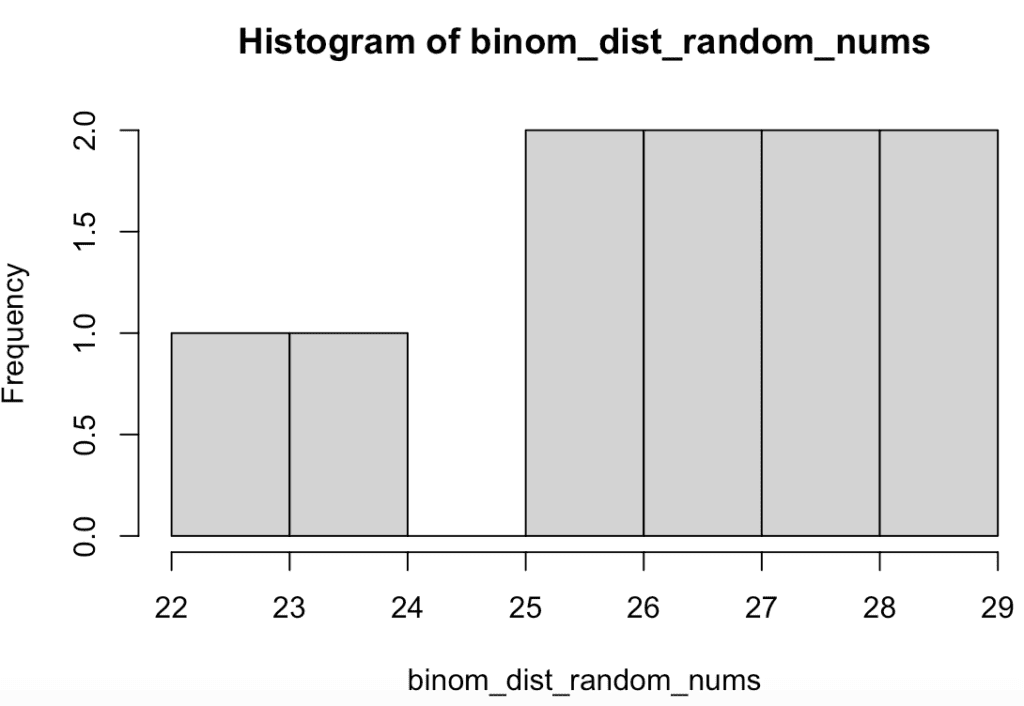# How to Generate Binomial Random Numbers in R

To generate binomial random numbers in R, you can use the “rbinom()” function. The rbinom() function in R is “used to generate a vector of binomial distributed random variables”.

The rbinom() function accepts the following three arguments.

1. n: It is the number of trials.
2. size: It is the number of successes.
3. prob: It is the probability of success for each trial.

Binomial distribution has two possible outcomes in statistics.

1. Success
2. Failure

The probability of success (p) and failure (1 – p) are fixed and do not change from one trial to the next. The number of successes (x) in a given number of trials (n) follows a binomial distribution.

A binomial random number is a random variable that follows a binomial distribution.

## Example 1

Generate 10 binomial distributed random numbers using the rbinom() function.

``````set.seed(99)

binom_dist_random_nums <- rbinom(10, size = 1, prob = 0.5)

print(binom_dist_random_nums)``````

Output

``  1 0 1 1 1 1 1 0 0 0``

We get an output of 10 random numbers with 0 and 1 values. A value of 1 suggests success, and a value of 0 suggests failure.

In this example, we defined a probability of 0.5, which means the output has a minimum of five success outcomes. To increase the success rate of the binomial distribution, increase the probability rate.

## Example 2

To create a different number of trials and probability of success,  change the values of the size and prob arguments.

``````set.seed(99)

binom_dist_random_nums <- rbinom(10, size = 30, prob = 0.9)

print(binom_dist_random_nums)``````

Output

`` 27 29 26 22 27 24 26 28 28 29``

In this example, we generated 10 binomial random numbers with a probability of success of 0.9 and 30 trials.

## Example 3

To create a histogram in R, you can use the “hist()” function and provide the binomial random numbers.

``````set.seed(99)

binom_dist_random_nums <- rbinom(10, size = 30, prob = 0.9)

hist(binom_dist_random_nums)``````

OutputThat’s it.